CUET  >  CAT Previous Year Questions: Number Series

# CAT Previous Year Questions: Number Series - General Test Preparation for CUET

## Introduction

The CAT Quantitative Ability (QA) Section places a significant emphasis on Number Systems, deeming it a pivotal topic. A robust grasp of the fundamentals of CAT Number Systems is crucial, necessitating active engagement in practice. Additionally, explore Number System questions from preceding CAT papers, complemented by detailed video solutions. This article delves into specific Number System questions pertinent to the CAT Exam. For CAT aspirants gearing up for one of India's most competitive management entrance exams, excelling in each section is of utmost importance.
This blog concentrates on CAT preparation, specifically honing in on the Number Systems segment. It offers a thorough review of questions from 2017 to 2022, providing step-by-step solutions and valuable insights to enhance your preparedness for success. Proficiency in Number Systems is imperative for CAT success, and this blog serves as a comprehensive resource for both novices and seasoned CAT aspirants.
It compiles past-year questions, expert tips, and strategies to aid you in mastering this challenging section. Join us on this CAT 2023 preparation journey as we dissect Number Systems, arming you with the knowledge and techniques necessary to conquer the CAT exam.

## Previous Year Questions

Q1: Question 1: If a, b, c and d are integers such that a + b + c + d = 30 then the minimum possible value of (a - b)2 + (a - c)2 + (a - d)2 is
Sol:
For the value of given expression to be minimum, the values of a, b, c and d should be as close as possible. 30/4 = 7.5
Since each one of these are integers so values must be 8, 8, 7, 7.
On putting these values in the given expression, we get
(8 - 8)2 + (8 - 7)2 + (8 - 7)
⇒ 1 + 1 = 2

Q2: If the product of three consecutive positive integers is 15600 then the sum of the squares of these integers is
(a) 1777
(b) 1785
(c) 1875
(d) 1877
Ans:
(d)
Sol: (x-1)x(x+1) = 15600
⇒ x3 - x = 15600
The nearest cube to 15600 is 15625 = 253
We can verify that x = 25 satisfies the equation above.
Hence the three numbers are 24, 25, 26. Sum of their squares = 1877

Q3: While multiplying three real numbers, Ashok took one of the numbers as 73 instead of 37. As a result, the product went up by 720. Then the minimum possible value of the sum of squares of the other two numbers is
Sol:
We know that one of the 3 numbers is 37.
Let the product of the other 2 numbers be x.
It has been given that 73x-37x = 720
36x = 720
x = 20
Product of 2 real numbers is 20.
We have to find the minimum possible value of the sum of the squares of the 2 numbers.
Let x=a*b
It has been given that a*b=20
The least possible sum for a given product is obtained when the numbers are as close to each other as possible.
Therefore, when a=b, the value of a and b will be √20.
Sum of the squares of the 2 numbers 20 + 20 = 40.
Therefore, 40 is the correct answer.

Q4: The number of integers x such that 0.25 ≤ 2x ≤ 200 and 2x + 2 is perfectly divisible by either 3 or 4, is
Sol:
At x = 0, 2x = 1 which is in the given range [0.25, 200]
2+ 2 = 1 + 2 = 3 Which is divisible by 3. Hence, x 0 is one possible solution.
At x = 1, 2x = 2 which is in the given range [0.25, 200]
2+ 2 = 2 + 2 = 3 Which is divisible by 4. Hence, x = 1 is one possible solution.
At x = 2, 2x = 4 which is in the given range [0.25, 200]
2x + 2 = 4 + 2 = 6 Which is divisible by 3. Hence, x = 2 is one possible solution.
At x = 3, 2x = 8 which is in the given range [0.25, 200]
2x + 2 = 8 + 2 = 3 Which is not divisible by 3 or 4. Hence, x = 3 can't be a solution.
At x = 4, 2x = 16 which is in the given range [0.25, 200]
2x + 2 = 16 + 2 = 18 Which is divisible by 3. Hence, x 4 is one possible solution.
At x = 5, 2x = 32 which is in the given range [0.25, 200]
2x + 2 = 32 + 2 = 34 Which is not divisible by 3 or 4. Hence, x = 5 can't be a solution.
At x = 6, 2= 64 which is in the given range [0.25, 200]
2x + 2 = 64 + 2 = 66 Which is divisible by 3. Hence, x 6 is one possible solution.
At x = 7, 2= 128 which is in the given range [0.25, 200]
2x + 2 = 128 + 2 = 130 Which is not divisible by 3 or 4. Hence, x = 7 can't be a solution.
At x = 8, 2= 256 which is not in the given range [0.25, 200]. Hence, x can't take any value greater than 7.
Therefore, all possible values of x = {0,1,2,4,6). Hence, we can say that 'x' can take 5 different integer values.

Q5: If the sum of squares of two numbers is 97, then which one of the following cannot be their product?
(a) -32
(b) 16
(c) 48
(d) 64
Ans:
(d)
Sol: Let 'a' and 'b' are those two numbers.
⇒ a2 + b2 = 97
⇒ a2 + b- 2ab = 97-2ab
⇒ (ab)= 97-2ab
We know that (a - b) ≥ 0
⇒ 97 - 2ab0
⇒ ab ≤ 48.5
Hence, ab ≠ 64. Therefore, option D is the correct answer.

Q6: The smallest integer n for which 4n > 1719 holds, is closest to
(a) 37
(b) 35
(c) 33
(d) 39
Ans:
(d)
Sol:
4n > 1719
16n/2 > 1719
Therefore, we can say that n/2 > 19
n > 38
Hence, option D is the correct answer.

Q7: What is the largest positive integer n such that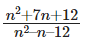is also a positive integer?
(a) 6
(b) 16
(c) 8
(d) 12
Ans:
(d)
Sol: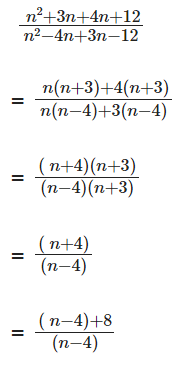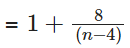which will be maximum when n - 4 = 8
n = 12

Q8: How many pairs (m, n) of positive integers satisfy the equation m2 + 105 = n2?
Sol:
n2 - m2 = 105
(n - m)(n + m) = 1*105, 3*35, 5*21, 7*15, 15*7, 21*5, 35*3, 105*1.
n - m = 1, n + m = 105  ⇒ n = 53, m = 52
n - m = 3, n + m = 35 ⇒ n = 19, m = 16
n - m = 5, n + m = 21  ⇒ n =13, m = 8
n - m = 7, n + m = 15 ⇒ n = 11, m = 4
n - m = 15, n + m = 7 ⇒ n = 11, m = -4
n - m = 21, n + m = 5 ⇒ n = 13, m = -8
n - m = 35, n + m = 3 ⇒ n = 19, m = -16
n - m = 105, n + m = 1 ⇒ n = 53, m = -52
Since only positive integer values of m and n are required. There are 4 possible solutions.

Q9: How many factors of 24 × 35 × 104 are perfect squares which are greater than 1?
Sol: 24 × 35 × 10
=24 × 35 × 24 × 5
= 2× 35 × 5
For the factor to be a perfect square, the factor should be even power of the number.
In 28, the factors which are perfect squares are 20, 22, 24, 26, 28 = 5
Similarly, in 35, the factors which are perfect squares are 30, 32, 34 = 3
In 54, the factors which are perfect squares are 50, 52, 54 = 3
Number of perfect squares greater than 1 = 5 × 3 × 3 - 1
= 44

Q10: In a six-digit number, the sixth, that is, the rightmost, digit is the sum of the first three digits, the fifth digit is the sum of first two digits, the third digit is equal to the first digit, the second digit is twice the first digit and the fourth digit is the sum of fifth and sixth digits. Then, the largest possible value of the fourth digit is
Sol:
Let the six-digit number be ABCDEF
F = A + B + C, E= A + B, C = A, B = 2A, D = E + F.
Therefore D = 2A + 2B + C = 2A + 4A + A = 7A.
A cannot be 0 as the number is a 6 digit number.
A cannot be 2 as D would become 2 digit number.
Therefore A is 1 and D is 7.

Q11: The product of two positive numbers is 616. If the ratio of the difference of their cubes to the cube of their difference is 157 : 3, then the sum of the two numbers is
(a) 58
(b) 85
(c) 50
(d) 95
Ans: (c)
Sol: Assume the numbers are a and b, then ab = 616
We have,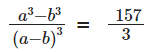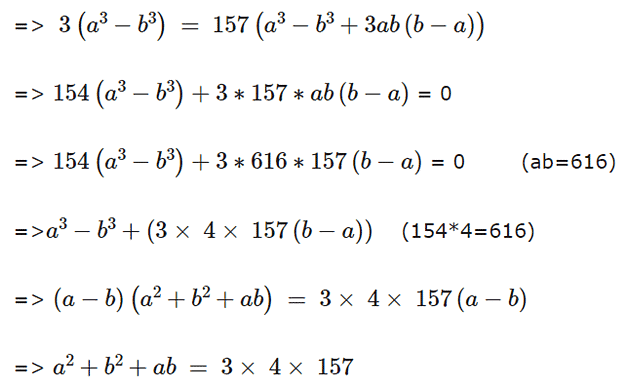Adding ab = 616 on both sides, we get
a2 + b2 + ab + ab = 3 × 4 × 157 + 616
=> (a + b)2 = 3 × 4 × 157 + 616 = 2500
=> a + b = 50

Q12: How many 3-digit numbers are there, for which the product of their digits is more than 2 but less than 7?
Sol: Let the number be ‘abc’. Then, 2 < a × b × c < 7. The product can be 3, 4, 5, 6.
We can obtain each of these as products with the combination 1,1, x where x = 3, 4, 5, 6.
Each number can be arranged in 3 ways, and we have 4 such numbers: hence, a total of 12 numbers fulfilling the criteria.
We can factories 4 as 2*2 and the combination 2,2,1 can be used to form 3 more distinct numbers.
We can factorize 6 as 2*3 and the combination 1,2,3 can be used to form 6 additional distinct numbers.
Thus a total of 12 + 3 + 6 = 21 such numbers can be formed.

Q13: The mean of all 4-digit even natural numbers of the form ‘aabb’,where a > 0, is
(a) 4466
(b) 5050
(c) 4864
(d) 5544
Ans:
(d)
Sol: The four digit even numbers will be of form:
1100, 1122, 1144 … 1188, 2200, 2222, 2244 … 9900, 9922, 9944, 9966, 9988
Their sum ‘S’ will be (1100 + 1100 + 22 + 1100 + 44 + 1100 + 66 + 1100 + 88) + (2200 + 2200 + 22 + 2200 + 44 +…)….+ (9900 + 9900 + 22 + 9900 + 44 + 9900 + 66 + 9900 + 88)
S=1100*5 + (22+44+66+88)+2200*5+(22 + 44 + 66 + 88)….+ 9900*5 + (22 + 44 + 66 + 88)
S=5*1100(1 + 2 + 3 + …9)+9(22 + 44 + 66 + 88)
S=5*1100*9*10/2 + 9*11*20
Total number of numbers are 9*5=45
Mean will be S/45 = 5*1100 + 44 = 5544.

Q14: A school has less than 5000 students and if the students are divided equally into teams of either 9 or 10 or 12 or 25 each, exactly 4 are always left out. However, if they are divided into teams of 11 each, no one is left out. The maximum number of teams of 12 each that can be formed out of the students in the school is
Sol: Since the total number of students, when divided by either 9 or 10 or 12 or 25 each, gives a remainder of 4, the number will be in the form of LCM(9,10,12,25)k + 4 = 900k + 4.
It is given that the value of 900k + 4 is less than 5000.
Also, it is given that 900k + 4 is divided by 11.
It is only possible when k = 2 and total students = 1804.
So, the number of 12 students group = 1800/12 = 150.

Q15: Consider six distinct natural numbers such that the average of the two smallest numbers is 14, and the average of the two largest numbers is 28. Then, the maximum possible value of the average of these six numbers is
(a) 23
(b) 24
(c) 23.5
(d) 22.5
Ans: (d)
Sol: Let the six numbers be a, b, c, d, e, f in ascending order
a + b = 28
e + f =  56
If we want to maximise the average then we have to maximise the value of c and d and maximise e and minimise f
e + f = 56
As e and f are distinct natural numbers so possible values are 27 and 29
Therefore c and d will be 25 and 26 respecitively
So average =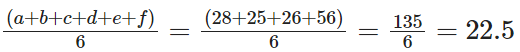Q16: For some natural number n, assume that (15,000)! is divisible by (n!)!. The largest possible value of n is
(a) 4
(b) 7
(c) 6
(d) 5
Ans: (b)
Sol: To find the largest possible value of n, we need to find the value of n such that n! is less than 15000.
7! = 5040
8! = 40320 > 15000
This implies 15000! is not divisible by 40320!
Therefore, maximum value n can take is 7.

Q17: How many 4-digit numbers, each greater than 1000 and each having all four digits distinct, are there with 7 coming before 3?
Sol: Here there are two cases possible
Case 1: When 7 is at the left extreme
In that case 3 can occupy any of the three remaining places and the remaining two places can be taken by (0,1,2,4,5,6,8,9)
So total ways 3(8)(7)= 168
Case 2: When 7 is not at the extremes
Here there are 3 cases possible. And the remaining two places can be filled in 7(7) ways.(Remember 0 can’t come on the extreme left)
Hence in total 3(7)(7)=147 ways
Total ways 168+147=315 ways

Q18: How many of the integers 1, 2, … , 120, are divisible by none of 2, 5 and 7?
(a) 42
(b) 41
(c) 40
(d) 43
Ans:
(b)
Sol: The number of multiples of 2 between 1 and 120 = 60
The number of multiples of 5 between 1 and 120 which are not multiples of 2 = 12
The number of multiples of 7 between 1 and 120 which are not multiples of 2 and 5 = 7
Hence, number of the integers 1, 2, … , 120, are divisible by none of 2, 5 and 7 = 120 – 60 – 12 – 7 = 41

Q19: Let N, x and y be positive integers such that N = x + y, 2 < x < 10 and 14 < y < 23. If N > 25, then how many distinct values are possible for N?
Sol:
Possible values of x = 3,4,5,6,7,8,9
When x = 3, there is no possible value of y
When x = 4, the possible values of y = 22
When x = 5, the possible values of y=21,22
When x = 6, the possible values of y = 20.21,22
When x = 7, the possible values of y = 19,20,21,22
When x = 8, the possible values of y=18,19,20,21,22
When x = 9, the possible values of y=17,18,19,20,21,22
The unique values of N = 26,27,28,29,30,31

Q20: How many integers in the set {100, 101, 102, …, 999} have at least one digit repeated?
Sol:
Total number of numbers from 100 to 999 = 900
The number of three digits numbers with unique digits:
_ _ _
The hundredth’s place can be filled in 9 ways ( Number 0 cannot be selected)
Ten’s place can be filled in 9 ways
One’s place can be filled in 8 ways
Total number of numbers = 9*9*8 = 648
Number of integers in the set {100, 101, 102, …, 999} have at least one digit repeated = 900 – 648 = 252

The document CAT Previous Year Questions: Number Series | General Test Preparation for CUET is a part of the CUET Course General Test Preparation for CUET.
All you need of CUET at this link: CUET

## General Test Preparation for CUET

176 videos|328 docs|399 tests

## FAQs on CAT Previous Year Questions: Number Series - General Test Preparation for CUET

 1. What is the importance of number series in the CAT exam?Ans. Number series questions are an integral part of the CAT exam. They test a candidate's ability to identify the pattern or sequence in a given set of numbers. These questions assess the candidate's logical reasoning and analytical skills, which are crucial for success in the exam. Practicing number series questions can help improve problem-solving abilities and enhance overall performance in the CAT exam.
 2. How can I improve my skills in solving number series questions?Ans. To improve skills in solving number series questions, it is important to practice regularly. Start by understanding the basic number patterns such as arithmetic progression, geometric progression, and Fibonacci series. Familiarize yourself with different types of patterns such as alternating patterns, increasing or decreasing patterns, or patterns involving prime numbers. Solve a variety of number series questions from previous year papers and mock tests to gain confidence and improve speed. Additionally, learn and apply techniques such as difference method, ratio method, and square method to solve complex number series questions efficiently.
 3. Are there any specific strategies to approach number series questions in the CAT exam?Ans. Yes, there are specific strategies that can help approach number series questions in the CAT exam. Firstly, carefully observe the given set of numbers and try to identify any patterns or sequences. Look for common differences, ratios, or any other mathematical relationships among the numbers. If a pattern is not immediately apparent, try to analyze the differences between the numbers. Secondly, work systematically by eliminating options that do not fit the identified pattern and narrowing down the possible answers. Finally, if you are unable to identify a pattern, make an educated guess by looking for clues in the options or using logical reasoning.
 4. How much time should I allocate to solve number series questions in the CAT exam?Ans. The time allocation for solving number series questions in the CAT exam depends on the difficulty level and your proficiency in solving such questions. As a general guideline, aim to spend around 1-2 minutes per question. It is advisable to quickly scan through the options and identify any patterns or relationships before diving into the calculations. If you are unable to identify a pattern within a reasonable timeframe, it is better to move on to the next question and come back to it later if time permits.
 5. Can I expect number series questions in other MBA entrance exams apart from CAT?Ans. Yes, number series questions are commonly asked in various MBA entrance exams apart from CAT. These exams include XAT, NMAT, SNAP, CMAT, and IIFT, among others. The level of difficulty and the types of number series questions may vary across different exams. Therefore, it is important to practice a wide range of number series questions from previous year papers and mock tests of these exams to familiarize yourself with the question patterns and enhance your problem-solving skills.

## General Test Preparation for CUET

176 videos|328 docs|399 testsExplore Courses for CUET examSignup to see your scores go up within 7 days! Learn & Practice with 1000+ FREE Notes, Videos & Tests.
10M+ students study on EduRev
Track your progress, build streaks, highlight & save important lessons and more!
Related Searches

,

,

,

,

,

,

,

,

,

,

,

,

,

,

,

,

,

,

,

,

,

;